# AP Computer Science A : Traversals

## Example Questions

### Example Question #1 : Computer Science

Consider the code below:

public static class BTNode {

public static final int PARSE_IN = 1;

public static final int PARSE_PRE = 2;

public static final int PARSE_POST = 3;

String name;

BTNode lPointer,rPointer;

public BTNode(String s) {

name = s;

lPointer = rPointer = null;

}

public void insert(String s) {

insert(this,s);

}

private static void insert(BTNode node,String s) {

int comparison = s.compareTo(node.name);

if(comparison < 0) {

if(node.lPointer != null) {

insert(node.lPointer,s);

} else {

node.lPointer = new BTNode(s);

}

} else if(comparison > 0) {

if(node.rPointer != null) {

insert(node.rPointer,s);

} else {

node.rPointer = new BTNode(s);

}

}

}

public ArrayList<String> parse(final int parseOrder) {

return parse(this,parseOrder);

}

private static ArrayList<String> parse(BTNode node, final int parseOrder) {

ArrayList<String> retVal = new ArrayList<String>();

if(node == null) {

return(retVal);

}

ArrayList<String> leftList = parse(node.lPointer,parseOrder);

ArrayList<String> rightList = parse(node.rPointer,parseOrder);

if(parseOrder == PARSE_PRE) {

} else if (parseOrder == PARSE_POST) {

} else {

}

return retVal;

}

}

public static void main(String[] args) {

String[] names = {"Hervaeus","Peter Auriol","Guiral","Felix","Lila","Lola","Yippy","Yiiiipppy","Acton","Pierce","Betty"};

BTNode node = new BTNode(names);

for(int i = 1; i < names.length; i++) {

node.insert(names[i]);

}

ArrayList<String> traversedNames = node.parse(BTNode.PARSE_IN);

for(String s : traversedNames) {

System.out.println(s);

}

}

What is the output for this method?

Hervaeus

Guiral

Felix

Acton

Betty

Peter Auriol

Lila

Lola

Yippy

Yiiiipppy

Pierce

Acton

Betty

Felix

Guiral

Hervaeus

Lila

Lola

Peter Auriol

Pierce

Yiiiipppy

Yippy

Peter Auriol

Hervaeus

Guiral

Acton

Betty

Felix

Lila

Lola

Yippy

Pierce

Yiiiipppy

Betty

Acton

Felix

Guiral

Lola

Lila

Pierce

Yiiiipppy

Yippy

Peter Auriol

Hervaeus

There is an error in the recursion in BTNode.

Acton

Betty

Felix

Guiral

Hervaeus

Lila

Lola

Peter Auriol

Pierce

Yiiiipppy

Yippy

Explanation:

The code given is a standard implementation of a binary tree containing an insert and a parse method.  For now, you can merely pay attention to your parse logic, particularly just the logic that will be invoked for the indicator value PARSE_IN.  (Notice that this is merely in the else of the parse method.  This is a "catch all" / default in case a bad value is given for the parse order.)

This is just the standard binary tree style of parsing based on our insert.  The smaller items are on the left of the current node, and the larger ones are on the right of it.  Thus, you have:

List of all smaller values   +   This current value    +    List of all larger values

Recursively, this will end with an ordered list, which is just what you need.

### Example Question #1 : Traversals

Consider the following code:

import java.util.ArrayList;

public class MethodClass5 {

public static class BTNode {

public static final int PARSE_IN = 1;

public static final int PARSE_PRE = 2;

public static final int PARSE_POST = 3;

String name;

BTNode lPointer,rPointer;

public BTNode(String s) {

name = s;

lPointer = rPointer = null;

}

public void insert(String s) {

insert(this,s);

}

private static void insert(BTNode node,String s) {

int comparison = s.compareTo(node.name);

if(comparison < 0) {

if(node.lPointer != null) {

insert(node.lPointer,s);

} else {

node.lPointer = new BTNode(s);

}

} else if(comparison > 0) {

if(node.rPointer != null) {

insert(node.rPointer,s);

} else {

node.rPointer = new BTNode(s);

}

}

}

public ArrayList<String> parse(final int parseOrder) {

return parse(this,parseOrder);

}

private static ArrayList<String> parse(BTNode node, final int parseOrder) {

ArrayList<String> retVal = new ArrayList<String>();

if(node == null) {

return(retVal);

}

ArrayList<String> leftList = parse(node.lPointer,parseOrder);

ArrayList<String> rightList = parse(node.rPointer,parseOrder);

if(parseOrder == PARSE_PRE) {

} else if (parseOrder == PARSE_POST) {

} else {

}

return retVal;

}

}

public static void main(String[] args) {

String[] names = {"Thomas Aquinas","Thomas Cajetan","Thomas Prufer","Thomas the Tank Engine","Thomas the Bread-Eater"};

BTNode node = new BTNode(names);

for(int i = 1; i < names.length; i++) {

node.insert(names[i]);

}

ArrayList<String> traversedNames = node.parse(BTNode.PARSE_POST);

for(String s : traversedNames) {

System.out.println(s);

}

}

}

What is the output for the main method above?

Thomas Aquinas

Thomas the Tank Engine

Thomas Prufer

Thomas Cajetan

Thomas the Tank Engine

Thomas Prufer

Thomas Cajetan

Thomas Aquinas

Thomas the Tank Engine

Thomas Prufer

Thomas Aquinas

Thomas Cajetan

Thomas Aquinas

Thomas Cajetan

Thomas Prufer

Thomas the Tank Engine

Thomas Aquinas

Thomas the Tank Engine

Thomas Prufer

Thomas Cajetan

Thomas the Tank Engine

Thomas Prufer

Thomas Cajetan

Thomas Aquinas

Explanation:

This code is a standard implementation of a Binary Tree class.  The call that we are making in main is meant to parse the tree (traverse it) in post-fix order.  This means that we will always look to the left of each node first, then to the right, then finally to the value we are at.  However, the tree is unbalanced, so the parsing will do much more right traveral than anything else.  See the following sequence of inserts:

Step 1:Step 2: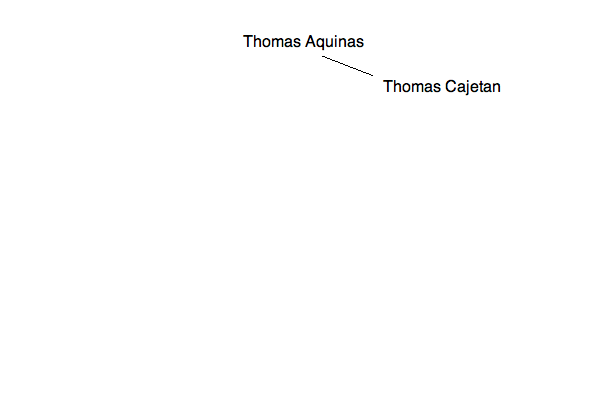Step 3: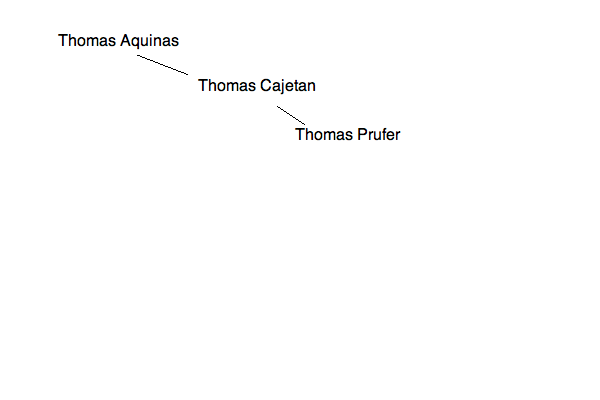Step 4: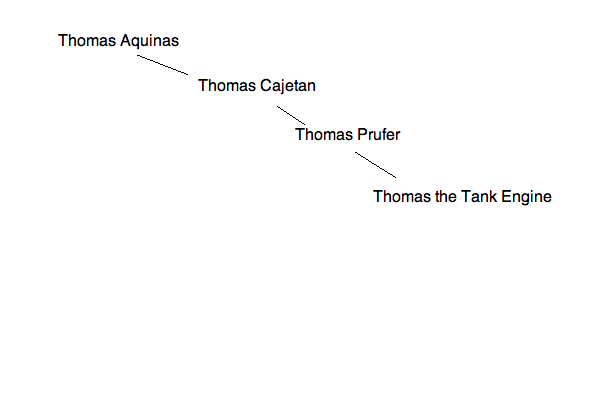Step 5: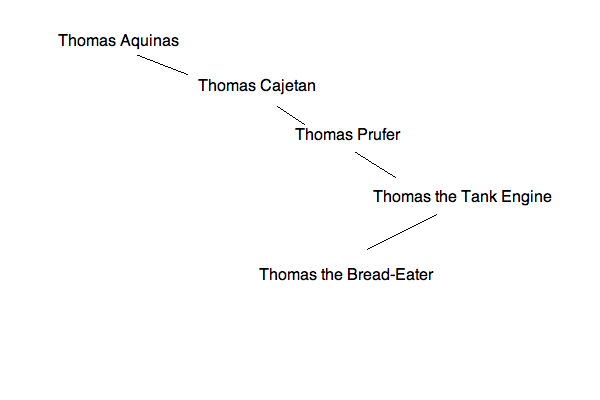Now, your traversal path will look like this: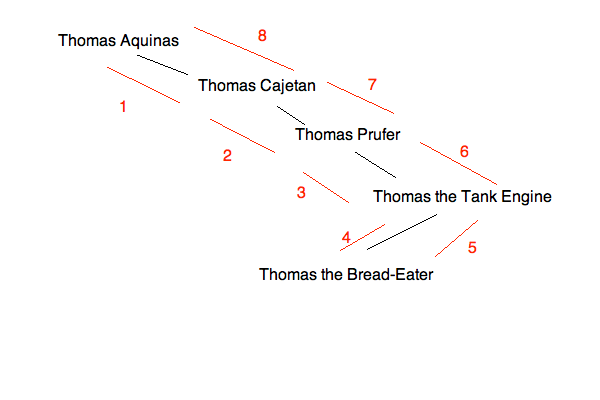Interestingly, this means that you will end up with a list that is in reverse order.  This is only the case for the given data as it happened to have been inserted.

### Example Question #1 : Traversals

The function recur is defined as follows:

public int recur(int x)
{
if (x <= 1)
{
return 1;
}
else
{
return x + recur(x/2);
}
}

How many times is recur called in the following declaration?

int num = recur(6);

5

4

3

2

1

3

Explanation:

The first call is in the declaration. Because 6 > 1, it calls recur, which makes the total 2.

Next, it calls recur(6/2). Because 3 > 1, it calls recur again, which makes the total 3.

Next, it calls recur(3/2). Because it's dividing integers, this evaluates to recur(1).

Because 1 <= 1, it doesn't call recur anymore, so that total number of calls is 3.

### Example Question #1 : Standard Operations & Algorithms

Which of the following code performs a multiplication by 5 of the elements of the array defined as:

int[][] vals = new int;

Presume that the array has been properly initialized and filled with values.

for(int i = 0; i < vals.length;i++) {

vals[i][0...99] *= 5;

}

for(int i = 0; i < vals.length;i++) {

for(int j = 0; j < vals[i].length; j++) {

vals[j][j] *= 5;

}

}

for(int i = 0; i < vals.length;i++) {

vals[i] *= 5;

}

for(int i = 0; i < vals.length;i++) {

for(int j = 0; j < vals[i].length; j++) {

vals[i][j] *= 5;

}

}

for(int i = 0; i < vals.length;i++) {

for(int j = 0; j < vals.length; j++) {

vals[i][j] *= 5;

}

}

for(int i = 0; i < vals.length;i++) {

for(int j = 0; j < vals[i].length; j++) {

vals[i][j] *= 5;

}

}

Explanation:

What we are looking for in this problem is a standard traversal of a 2D array.  When you do this, you need to make sure that you iterate through both the rows and the columns.  In order to do this, you first must set up a loop like:

for(int i = 0; i < vals.length;i++) {...

The value of vals.length indicates the number of rows in the 2D array.

Now, for each row, you have a certain number of columns.  (A 2D array is like an "array of arrays".)  Thus, for each row, you need to run through the entire set of columns for that row:

for(int j = 0; j < vals[i].length; j++) { ...

Notice that none of the incorrect questions use vals[i].length in this way.  Thus, none of them iterate through the two dimensions of the array correctly.

# TREE TRAVERSALS

Given the following tree structure: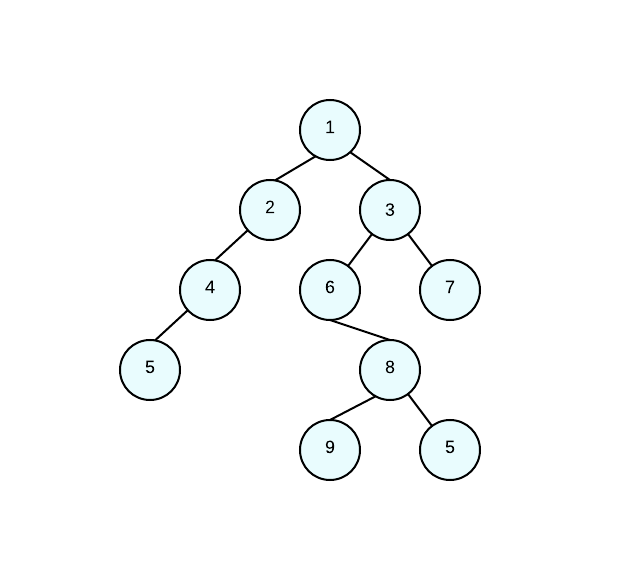What is the pre-order traversal of the tree?

1 2 4 5 3 6 8 9 5 7

1 2 3 4 5 5 6 7 8 9

5 4 2 1 3 6 7 8 9 5

1 2 3 4 6 7 5 8 9 5

1 2 4 5 3 6 8 9 5 7

Explanation:

When a preorder traversal is done, you go through the following:

1. ROOT

2. LEFT CHILD

3. RIGHT CHILD

Therefore, starting at the root (1), we go to the LEFT node (2). That node however, is the root/parent of other nodes, so we go left again (4). That node is a parent/root, so we go left (5). Now it's time to go to the right; however, only node 1 has a right child, so going to the right of one we land at (3). That node is the parent/root of more children so we go left (6). Node 6 doesn't have any left children, but it does have a right so we go right (8). Node 8 has a left child to traverse (9) and then a right (5). And we finish the traversal by going to the right of three (7). This is a rather complicated example; however, make sure you can traverse the tree properly.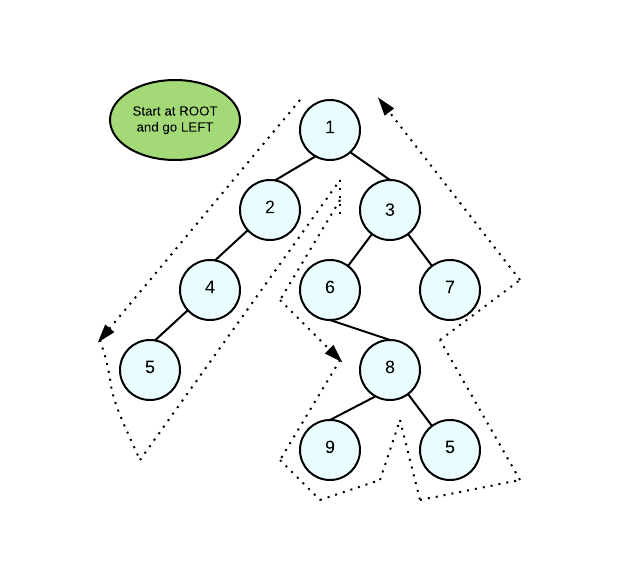In the above image, you can see the approach of the pre-order traversal. First you go through the roots, then the left trees, then the right trees. Make sure that if you're guiding yourself by the image above, that you don't repeat the nodes that you already traversed. Understanding the algorithm is key.

### Example Question #1 : Standard Operations & Algorithms

Traverse and print out this list

List<Integer> integers = new ArrayList<Integer>();

for (int i = 0; i < integers.size()-1; i++) {

System.out.println(integers[i]);

}

for (int i = 0; i < integers.length-1; i++) {

System.out.println(integers.get(i));

}

System.out.println(integers.get(0));

System.out.println(integers.get(1));

System.out.println(integers.get(2));

for (int i = 0; i < integers.size()-1; i++) {

System.out.println(integers.get(i));

}

for (int i = 0; i < integers.size()-1; i++) {

System.out.println(integers.get(i));

}

Explanation:

Use a for loop to traverse the list. The .size() method is used for Lists as opposed to the .length method for arrays. The .get() method is used for Lists as opposed to accessing the index for arrays.

### Example Question #1 : Traversals

Suppose you are given an array of integers:

int array[] = {1,2,3,4,5};

and the following method:

public static void printArray(int[] arr)

{

for (int a = 0; r < arr.length-1; a++)

{

if (a%2==0)

{

System.out.println(arr[a]);

}

}

}

After the method cacll printArray(array) is called, the output would be:

2 4 6

1

3

1

3

5

2

4

1

2

3

4

5

1

3

Explanation: